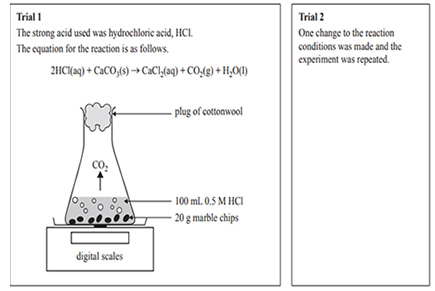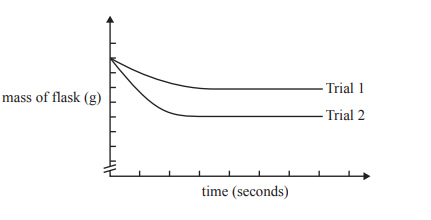Rates (2016 VCE) 1) A student set up an experiment to test the effect of different factors on the rate and extent of the reaction between a strong acid and marble chips (calcium carbonate, CaCO3). In each trial, the mass of the flask and its contents was measured every 30 seconds, from the instant the reactants were mixed.The results of the two trials were graphed on the same axes and are shown belowIn Trial 2, the student must have A. heated the 0.5 M HCl before adding it to the flask. B. doubled the volume of 0.5 M HCl added to the flask. C. used 100 mL of 0.5 M H2SO4 instead of 100 mL of 0.5 M HCl. D. used the same mass of marble but crushed it into a powder. Solution